# On the influence of the seed graph in the preferential attachment modelThe preferential attachment model, introduced in 1992 by Mahmoud and popularized in 1999 by Barabási and Albert, has attracted a lot of attention in the last decade. In its simplest form it describes the evolution of a random tree. Formally we denote bythe preferential attachment tree on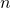vertices which is defined by induction as follows. Firstis the unique tree on two vertices. Then, given,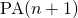is formed fromby adding a new vertex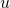and a new edge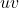where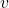is selected at random among vertices inaccording to the following probability distribution:where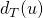denotes the degree of vertexin a tree. In other words vertices of large degrees are more likely to attract the new nodes. This model of evolution is argued to be a good approximation for things such a network of citations, or the internet network.

One of the main reason for the success of the preferential attachment model is the following theorem, which shows that the degree distribution infollows a power-law, a feature that many real-world networks (such as the internet) exhibit but which is not reproduced by standard random graph models such as the Erdös-Rényi model.

Theorem [Bollobás, Riordan, Spencer and Tusnády (2001)Let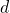be fixed. Then as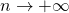, the proportion of vertices with degreetends in probability toWhile the above theorem is a fine and interesting mathematical result, I do not view it as the critical aspect of the preferential attachment model (note that Wikipedia disagrees). In my opinionis simply interesting merely because of its natural rule of evolution.

Now think about the application of the PA model to the internet. Of course there is a few obvious objections, such as the fact that ina website can only link to one other website. While this is clearly ridiculous I think thatstill contains the essence of what one would like to capture to model the evolution of the internet. However there is one potentially important aspect which is overlooked: in the early days of the internet the PA model was probably very far from being a good approximation to the evolution of the network. It is perhaps reasonable to assume that after 1995 the network was evolving according to PA, but certainly from 1970 to 1995 the evolution followed fundamentally different rules. This observation suggests to study the preferential attachment model with a seed.

Thus we are now interested in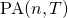, whereis a finite seed tree. Formallyis also defined by induction, where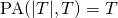andis formed fromas before. A very basic question which seems to have been overlooked in the literature is the following: what is the influence of the seedonasgoes to infinity?

In our recent joint work with Elchanan Mossel and Miklos Racz we looked exactly at this question. More precisely we ask the following: given two seed treesand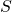, do the distributionsandremain separated (say in total variation distance) asgoes to infinity? In other words we are interested in the following quantity:A priori it could be thatfor anyand, which would mean that the seed has no influence and that the preferential attachment “forgets” its initial conditions. We prove that this is far from true:

Theorem [Bubeck, Mossel and Racz (2014)] Letandbe two finite trees on at least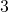vertices. If the degree distributions inandare different, then.

If I wanted to make a bold statement I could say that this theorem implies the following: by looking at the internet network today, one can still “see” the influence of the topological structure of the internet back in the 90’s. In other words to a certain extent one can go back in time and potentially infer some properties that people may have believed to be lost (perhaps some army’s secrets hidden in the structure of the ARPANET?). Of course at this stage this is pure science fiction, but the theorem certainly leaves that possibility open. Note that we believe that the theorem can even be strengthen to the following statement:

Conjecture Letandbe two finite trees on at leastvertices. Ifandare non-isomorphic, then.

These statements show that even whenis large one can still “see” inthe influence of the original seed. However by considering the total variation distance we allow global statistics that depend on entire tree. What about local statistics that could be computed by an agent looking only at a finite neighborhood around her? Mathematically this question can be interpreted in the framework of the Benjamini-Schramm limit. Recall that a sequence of random graphs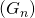tends to a random infinite rooted tree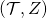(is the random root) if for any, the random ball of radiusaround a random vertexin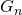tends in distribution to the random ball of radiusaroundin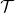. In other words whenis large enough a random agent cannot tell if she is inor inby looking at a finite neighborhood around her. One has the following theorem for the weak limit of the PA model:

Theorem [Berger, Borgs, Chayes and Saberi (2014)The Benjamini-Schramm limit ofis the Pólya-point graph with.

We extend this result to an arbitrary seed and show that locally the seed has no influence:

Theorem [Bubeck, Mossel and Racz (2014)For any treethe Benjamini-Schramm limit ofis the Pólya-point graph with.

Thus, while the army’s secret of the 90’s might be at risk if one looks at the overall topology of the current internet network, these secrets are safe from any local agent who would only access a (random) finite part of the network.

These new results on the PA model naturally lead to a host of new problems. We end the paper with a list of 7 open problems, I recommend to take a look at them (and try to solve them)!

This entry was posted in Random graphs. Bookmark the permalink.

## 9 Responses to "On the influence of the seed graph in the preferential attachment model"

••••••••